# Javascript Function: Intro

by Ridwan Fadilah, updated on Apr 04, 2020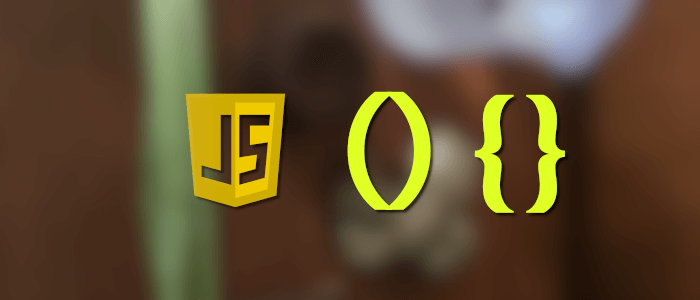#### Introducing, learning, and understanding about javascript function and function definition, usage, and example

“Functions are the very best part of JavaScript. It's where most of the power and the beauty of this language is.” - Douglas Crockford.

Javascript function is a subprogram/subroutine that can be called in other parts of the program. In other programming languages, we know a method, class, constructor, and module. In javascript, we can do all that just using a function.

Here's I'll try to show you a basic to use the function in javascript.

## Why do we need to use a function?

Before we learn more about javascript function, we must be knowing a few reasons why we should use a function.

It is because the javascript function is :

• Reusability (DRY: Do not Repeat Yourself). It means we no need to rewrite the code of a program. With function, we only need to use one block of a program that can use repeatedly.
• Decomposition / Abstraction. It means to hide the complexity of the program that we write.
• Modularity. When we hide the complexity with change the program to subprogram or modules, that can help us to find an error.

The 'function' divided into two categories. Built-in function and User-defined function.

A built-in function is a function has made by javascript like:

• confirm()
• prompt()
• and etc.

## Built-in function - string

It is some example Built-in function in javascript related to the string.

• charAt(), slice(), split(), toString(), toLowerCase(), toUpperCase(), and etc.

There so many of the functions in javascript that can use. I will show you the one of usage for an example built-in function that I have mentioned above.

Use charAt() to know what the character has in a string:

``````    <!DOCTYPE html>
<html lang="en">

<title>Function</title>

<body>

<script>
console.log(name.charAt(5));
</script>

</body>

</html>``````

It uses to know what the fifth character from the string we have.
Index starts from 0 (zero). Then the function above will produce "n" as the fifth character: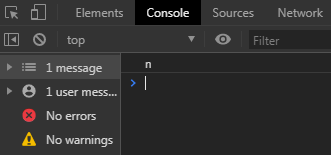## Built-in function - Math

It is some example Built-in function in javascript related to math :

• sin(), cos(), tan(), random(), round(), floor(), ceil(), log(), and etc.

Here's an example:

``````    <!DOCTYPE html>
<html lang="en">

<title>Function</title>

<body>

<script>
let num = Math.random();
console.log(num);
</script>

</body>

</html>``````

It will produce random numbers from 0 - 1 (zero to one) like this: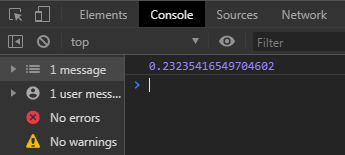That's a basics example of a built-in function. Next, we'll introduce the user-defined function.

## User-defined function

A user-defined function is a function created by the user. Before we learned, lets knowing some the following about this function:

• The function must contain the "function" keyword
• We can add a name of the function (optional)
• In this function, we can add a parameter/argument
• Function body place at { }. The body contains a set statement
• The function can return a value or not

To create a user-defined function are have two ways. First, using the function declaration or using the function expression.

That's some of the following about how to create a function by ourselves.

### Function Declaration

I'll make a program that uses a function declaration. For example, I want to create a program that calculates the sum from two numbers. The function will have a "function" keyword, function name, parameter, variable in the function body, and return a value from calculating the sum of numbers.

It's the structure of the function: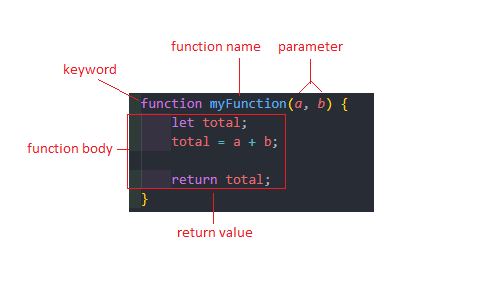Example:

``````    <!DOCTYPE html>
<html lang="en">

<title>Function</title>

<body>

<script>
function myFunction(a, b) {
let total;
total = a + b;

}

</script>

</body>

</html>``````

The browser will show a total of the sum from calculating the number in a popup window like this: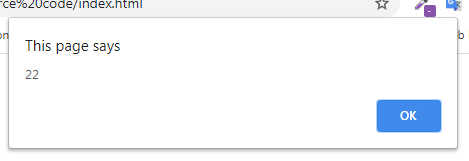Functions can be called repeatedly in one program.

Example:

``````    <!DOCTYPE html>
<html lang="en">

<title>Function</title>

<body>

<script>
function myFunction(a, b) {
let total;
total = a + b;

}

</script>

</body>

</html>``````

The browser will display three popups one by one: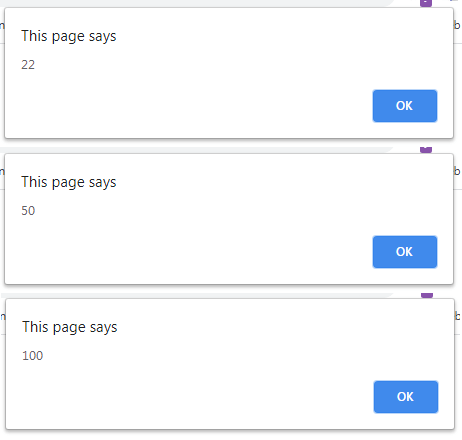### Function Expression

No many different from the function declaration, the differences are function expression is placed in a variable.

For example, I create a variable with the name like the function name that we use before, contains a "function" keyword, and returns a value.

Example:

``````    <!DOCTYPE html>
<html lang="en">

<title>Function</title>

<body>

<script>
let myFunction = function (a, b) {
let total;
total = a + b;

}

</script>

</body>

</html>``````

result: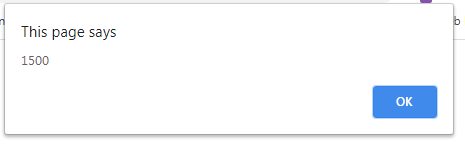That's just a basic tutorial to create and run a javascript function. I will show you more examples of the javascript function as soon as possible.

You can get the full source code from our GitHub.

That just the basic. If you need more deep learning about HTML, CSS, Javascript or related you can take the following cheap course:

• HTML & HTML5 For Beginners (A Practical Guide)• Web - HTML• Learn HTML, CSS, JAVASCRIPT• JavaScript:• Learn JavaScript Fundamentals• Learning JavaScript Shell Scripting